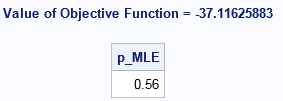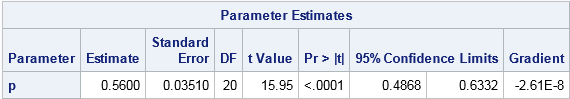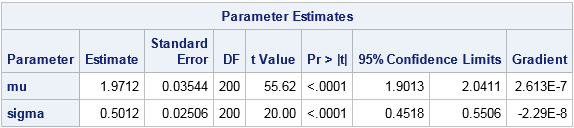In a previous article, I showed two ways to define a log-likelihood function in SAS. This article shows two ways to compute maximum likelihood estimates (MLEs) in SAS: the nonlinear optimization subroutines in SAS/IML and the NLMIXED procedure in SAS/STAT. To illustrate these methods, I will use the same data sets from my previous post. One data set contains binomial data, the other contains data that are lognormally distributed.

### Maximum likelihood estimates for binomial data from SAS/IML

I previously wrote a step-by-step description of how to compute maximum likelihood estimates in SAS/IML. SAS/IML contains many algorithms for nonlinear optimization, including the NLPNRA subroutine, which implements the Newton-Raphson method.

In my previous article I used the LOGPDF function to define the log-likelihood function for the binomial data. The following statements define bounds for the parameter (0 < p < 1) and provides an initial guess of p0=0.5:

```/* Before running program, create Binomial and LN data sets from previous post */   /* Example 1: MLE for binomial data */ /* Method 1: Use SAS/IML optimization routines */ proc iml; /* log-likelihood function for binomial data */ start Binom_LL(p) global(x, NTrials); LL = sum( logpdf("Binomial", x, p, NTrials) ); return( LL ); finish;   NTrials = 10; /* number of trials (fixed) */ use Binomial; read all var "x"; close;   /* set constraint matrix, options, and initial guess for optimization */ con = { 0, /* lower bounds: 0 < p */ 1}; /* upper bounds: p < 1 */ opt = {1, /* find maximum of function */ 2}; /* print some output */ p0 = 0.5; /* initial guess for solution */ call nlpnra(rc, p_MLE, "Binom_LL", p0, opt, con); print p_MLE;```The NLPNRA subroutine computes that the maximum of the log-likelihood function occurs for p=0.56, which agrees with the graph in the previous article. We conclude that the parameter p=0.56 (with NTrials=10) is "most likely" to be the binomial distribution parameter that generated the data.

### Maximum likelihood estimates for binomial data from PROC NLMIXED

If you've never used PROC NLMIXED before, you might wonder why I am using that procedure, since this problem is not a mixed modeling regression. However, you can use the NLMIXED procedure for general maximum likelihood estimation. In fact, I sometimes joke that SAS could have named the procedure "PROC MLE" because it is so useful for solving maximum likelihood problems.

PROC NLMIXED has built-in support for computing maximum likelihood estimates of data that follow the Bernoulli (binary), binomial, Poisson, negative binomial, normal, and gamma distributions. (You can also use PROC GENMOD to fit these distributions; I have shown an example of fitting Poisson data.)

The syntax for PROC NLMIXED is very simple for the Binomial data. You use the PARMS statement to supply an initial guess for the parameter p. On the MODEL statement, you declare that you want to model the X variable as Binom(p) where NTrials=10. Be sure to ALWAYS check the documentation for the correct syntax for a binomial distribution. Some functions like PDF, CDF, and RAND use the p parameter as the first argument: Binom(p, NTrials). Some procedure (like the MCMC and NLMIXED) use the p parameter as the second argument: Binom(NTrials, p).

```/* Method 2: Use PROC NLMIXED solve using built-in modeling syntax */ proc nlmixed data=Binomial; parms p = 0.5; * initial value for parameter; NTrials = 10; model x ~ binomial(NTrials, p); run;```Notice that the output from PROC NLMIXED contains the parameter estimate, standard error, and 95% confidence intervals. The parameter estimate is the same value (0.56) as was found by the NLPNRA routine in SAS/IML. The confidence interval confirms what we previously saw in the graph of the log-likelihood function: the function is somewhat flat near the optimum, so a 95% confidence interval is wide: [0.49, 0.63].

### Maximum likelihood estimates for lognormal data

You can use similar syntax to compute MLEs for lognormal data. The SAS/IML syntax is similar to the binomial example, so it is omitted. To view it, download the complete SAS program that computes these maximum likelihood estimates.

PROC NLMIXED does not support the lognormal distribution as a built-in distribution, which means that you need to explicitly write out the log-likelihood function and specify it in the GENERAL function on the MODEL statement. Whereas in SAS/IML you have to use the SUM function to sum the log-likelihood over all observations, the syntax for PROC NLMIXED is simpler. Just as the DATA step has an implicit loop over all observations, the NLMIXED procedure implicitly sums the log-likelihood over all observations. You can use the LOGPDF function, or you can explicitly write the log-density formula for each observation.

If you look up the lognormal distribution in the list of "Standard Definition" in the PROC MCMC documentation, you will see that one parameterization of the lognormal PDF in terms of the log-mean μ and log-standard-deviation σ is
f(x; μ, σ) = (1/(sqrt(2π σ x) exp(-(log(x)-μ)**2 / (2σ**2))
When you take the logarithm of this quantity, you get two terms, or three if you use the rules of logarithms to isolate quantities that do not depend on the parameters:

```proc nlmixed data=LN; parms mu 1 sigma 1; * initial values of parameters; bounds 0 < sigma; * bounds on parameters; sqrt2pi = sqrt(2*constant('pi')); LL = -log(sigma) - log(sqrt2pi*x) /* this term is constant w/r/t (mu, sigma) */ - (log(x)-mu)**2 / (2*sigma**2); /* Alternative: LL = logpdf("Lognormal", x, mu, sigma); */ model x ~ general(LL); run;```The parameter estimates are shown, along with standard errors and 95% confidence intervals. The maximum likelihood estimates for the lognormal data are (μ, σ) = (1.97, 0.50). You will get the same answer if you use the LOGPDF function (inside the comment) instead of the "manual calculation." You will also get the same estimates if you omit the term log(sqrt2pi*x) because that term does not depend on the MLE parameters.

In conclusion, you can use nonlinear optimization in the SAS/IML language to compute MLEs. This approach is especially useful when the computation is part of a larger computational program in SAS/IML. Alternatively, the NLMIXED procedure makes it easy to compute MLEs for discrete and continuous distributions. For some simple distributions, the log-likelihood functions are built into PROC NLMIXED. For others, you can specify the log likelihood yourself and find the maximum likelihood estimates by using the GENERAL function.

The post Two ways to compute maximum likelihood estimates in SAS appeared first on The DO Loop.